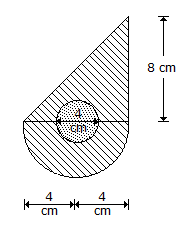# Civil Engineering - Applied Mechanics

### Exercise :: Applied Mechanics - Section 3

41.

A marble ball is rolled on a smooth floor of a room to hit a wall. If the time taken by the ball in returning to the point of projection is twice the time taken in reaching the wall, the coefficient of restitution between the ball and the wall, is

 A. 0.25 B. 0.5 C. 0.75 D. 1

Explanation:

No answer description available for this question. Let us discuss.

42.

A number of forces acting simultaneously on a particle of a body

 A. may not be replaced by a single force B. may be replaced by a single force C. may be replaced by a single force through C.G. of the body D. may be replaced by a couple E. none of these.

Explanation:

No answer description available for this question. Let us discuss.

43.

The moment of inertia of the shaded portion of the area shown in below figure about the X-axis, isA. 229.34 cm4 B. 329.34 cm4 C. 429.34 cm4 D. 529.34 cm4.

Explanation:

No answer description available for this question. Let us discuss.

44.

The force acting on a point on the surface of a rigid body may be considered to act

 A. at the centre of gravity of the body B. on the periphery of the body C. on any point on the line of action of the force D. at any point on the surface normal to the line of action of the force.

Explanation:

No answer description available for this question. Let us discuss.

45.

A satellite is said to move in a synchronous orbit if it moves at an altitude of 36, 000 km with a maximum velocity of about

 A. 7000 km per hour B. 8000 km per hour C. 9000 km per hour D. 10, 000 per hour E. 11, 000 km per hour.